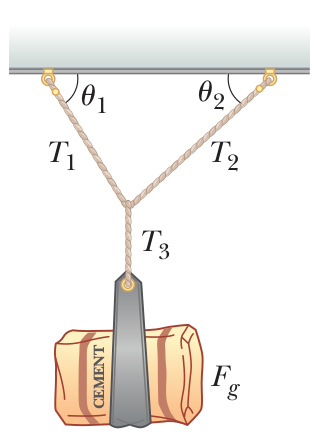# Problem: A bag of cement weighing Fg = 325 N hangs in equilibrium from three wires as shown in the figure. Two of the wires make angles θ1 = 60.0° and θ2 = 40.0° with the horizontal. Assuming the system is in equilibrium, find the tensions T1, T2, and T3 in the wires.

###### FREE Expert Solution

We're asked for the tension in each of the wires supporting the bag of cement.

This is a 2D Equilibrium type of problem with multiple forces in different angles. As usual for equilibrium problems, we'll follow these steps:

1. Draw a free body diagram for each point of interest
2. Set up our equilibrium equations
3. Solve for the target.

In two dimensions, we use equilibrium equations in component form:

$\overline{){\mathbf{\sum }}{{\mathbit{F}}}_{{\mathbit{x}}}{\mathbf{=}}{\mathbf{0}}\phantom{\rule{0ex}{0ex}}{\mathbf{\sum }}{{\mathbit{F}}}_{{\mathbit{y}}}{\mathbf{=}}{\mathbf{0}}}$

Anytime we're working with forces that aren't at 90° angles to each other, we'll also need some of the equations to convert magnitude-angle notation to components.

Magnitude: $\overline{)\mathbf{|}\stackrel{\mathbf{⇀}}{\mathbit{F}}\mathbf{|}{\mathbf{=}}\sqrt{{{\mathbit{F}}_{\mathbit{x}}}^{\mathbf{2}}\mathbf{+}{{\mathbit{F}}_{\mathbit{y}}}^{\mathbf{2}}}}$

Angle:

Components of a force:

84% (242 ratings)###### Problem Details

A bag of cement weighing Fg = 325 N hangs in equilibrium from three wires as shown in the figure. Two of the wires make angles θ1 = 60.0° and θ2 = 40.0° with the horizontal. Assuming the system is in equilibrium, find the tensions T1, T2, and T3 in the wires.